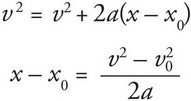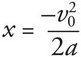# AP Physics 1 Question 200: Answer and Explanation

### Test Information

Question: 200

10. A car travelling at a speed of v0 applies its brakes, skidding to a stop over a distance of x m. Assuming that the deceleration due to the brakes is constant, what would be the skidding distance of the same car if it were traveling with twice the initial speed?

• A. 2x m
• B. 3x m
• C. 4x m
• D. 8x m

Explanation:

C

The variables involved in this question are the initial velocity (given in both cases), the acceleration (constant), final velocity (0 in both cases), and displacement (the skidding distance). As the missing variable is time, use Big Five #5 to solve for the displacement:As the initial position, x0, and the final velocity, v2, are equal to 0, this equation simplifies toWhen the initial velocity is doubled, the final position quadruples. (Note that the acceleration in this problem is negative, as the brakes cause the car to decelerate).